Test: Single Phase Full wave mid point converters

# Test: Single Phase Full wave mid point converters

Test Description

## 10 Questions MCQ Test Power Electronics | Test: Single Phase Full wave mid point converters

Test: Single Phase Full wave mid point converters for Electrical Engineering (EE) 2023 is part of Power Electronics preparation. The Test: Single Phase Full wave mid point converters questions and answers have been prepared according to the Electrical Engineering (EE) exam syllabus.The Test: Single Phase Full wave mid point converters MCQs are made for Electrical Engineering (EE) 2023 Exam. Find important definitions, questions, notes, meanings, examples, exercises, MCQs and online tests for Test: Single Phase Full wave mid point converters below.
Solutions of Test: Single Phase Full wave mid point converters questions in English are available as part of our Power Electronics for Electrical Engineering (EE) & Test: Single Phase Full wave mid point converters solutions in Hindi for Power Electronics course. Download more important topics, notes, lectures and mock test series for Electrical Engineering (EE) Exam by signing up for free. Attempt Test: Single Phase Full wave mid point converters | 10 questions in 30 minutes | Mock test for Electrical Engineering (EE) preparation | Free important questions MCQ to study Power Electronics for Electrical Engineering (EE) Exam | Download free PDF with solutions
 1 Crore+ students have signed up on EduRev. Have you?
*Answer can only contain numeric values
Test: Single Phase Full wave mid point converters - Question 1

### SCRs with a peak forward rating of 2 kV and an average on-state current rating of 50 A are used in a single-phase mid-point converter. If the factor of safety is 2.5, the power that can be handled by this converter is ______kW

Detailed Solution for Test: Single Phase Full wave mid point converters - Question 1

The maximum voltage across SCRs in mod point connection is 2 Vm. So the maximum voltage of SCR =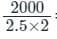= 400 V

The maximum average power that the midpoint converter can handle is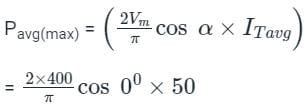= 12.732 kW

Test: Single Phase Full wave mid point converters - Question 2

### In a center tap full wave rectifier, if the peak voltage applied between the center tap and one of the secondary 200 V, then the reverse biased diode is applied with a maximum voltage of:

Detailed Solution for Test: Single Phase Full wave mid point converters - Question 2

Concept:

Peak inverse voltage: It is a voltage across the diode when it is reversed bias.

The following circuit is the center tapped full-wave rectifier.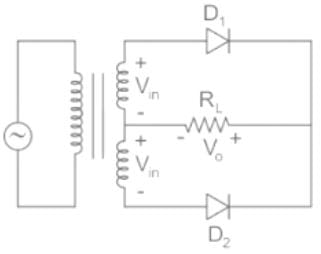Consider for positive half cycle, D1 is FB and D2 is reversed biased.

In loop 1, Apply KVL

⇒ V0 = Vin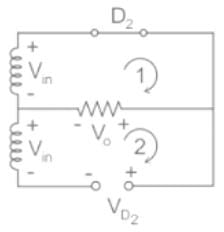Now, apply KVL in loop 2

Vin + V0 = VD2

⇒ VD2 = Vin + Vin = 2Vin

PIV of the centre tapped full wave rectifier = 2Vin

Calculation:

Given that, the center-tapped full-wave rectifier and Vin = 200 V (Peak) between the centre tap and one end of secondary.

Peak inverse voltage = 2Vin = 400 V

Test: Single Phase Full wave mid point converters - Question 3

### A full-wave rectifier uses 2 diodes. The internal resistance of each diode is 20 Ω. The transformer RMS secondary voltage from centre tap to each end of the secondary is 50 V and the load resistance is 980 Ω. Mean load current will be

Detailed Solution for Test: Single Phase Full wave mid point converters - Question 3

Concept:

Center tapped full wave rectifier:

• The Center tapped full-wave rectifier is a device used to convert the AC input voltage into DC voltage at the output terminals.
• It employs a transformer with the secondary winding tapped at the center point. And it uses only two diodes, which are connected to the opposite ends of a center-tapped transformer as shown in the figure below.
• The center tap is usually considered as the ground point or the zero voltage reference point.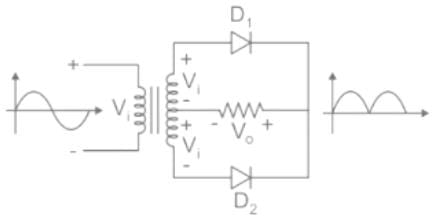Analysis: The DC output voltage or average output voltage can be calculated as follows,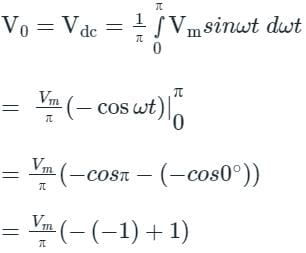V0 = 2Vm / π

Now we can calculate the average or mean current of load by dividing the average load voltage by load resistance RL. Therefore mean load current is given by

I0 = V0 / RL

If the internal resistance of the diode is given in that case mean load current I0 = V0 / (RL + r)

Where r = internal resistance of the diode.

Calculation:

Given that

Rms value of supply voltage V = 50 V

The internal resistance of diode r = 20 Ω

The load resistance RL = 980 Ω

Maximum voltage on the secondary side Vm = √2 V = √2 × 50 = 70.7 V

Average or DC output voltage V0 = (2 × 70.7) / π = 45 V

Average or mean load current is

I0 = V0 / (RL + r) = 45 /(980 + 20) = 45 mA

Test: Single Phase Full wave mid point converters - Question 4

In a two-diode full wave rectifier, with a load current requirement of 4.2 A, what should be the current ratings of the diodes used?

Detailed Solution for Test: Single Phase Full wave mid point converters - Question 4

Concept:

Center tapped full wave rectifier:

• The Center tapped full-wave rectifier is a device used to convert the AC input voltage into DC voltage at the output terminals.
• It employs a transformer with the secondary winding tapped at the center point. And it uses only two diodes, which are connected to the opposite ends of a center-tapped transformer as shown in the figure below.
• The center tap is usually considered as the ground point or the zero voltage reference point.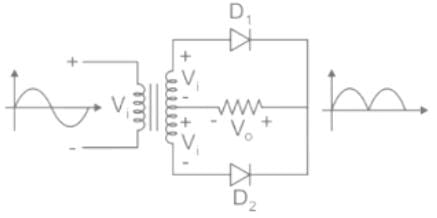Analysis:

The DC output voltage or average output voltage can be calculated as follows,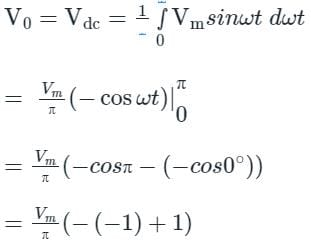V0 = 2Vm / π

Now we can calculate the average or mean current of load by dividing the average load voltage by load resistance RL. Therefore mean load current is given by

I0 = V0 / RL

Current through each diode will be half of the load current, as each diode conducts only for the half cycle.

Calculation:

Load current = I0 = 4.2 A

Current through each diode = I0 / 2 = 4.2 / 2 = 2.1 A

∴The current ratings of the each diode is 2.1 A.

Test: Single Phase Full wave mid point converters - Question 5

A single-phase mid-point full-wave SCR converter with maximum mid-point voltage of Vm volts develops an average output voltage across a resistive load at firing delay angles of 0 and π/2 rad., respectively, as

Detailed Solution for Test: Single Phase Full wave mid point converters - Question 5

A single-phase mid-point full-wave SCR converter:

In this circuit, two thyristors are used and are connected as shown below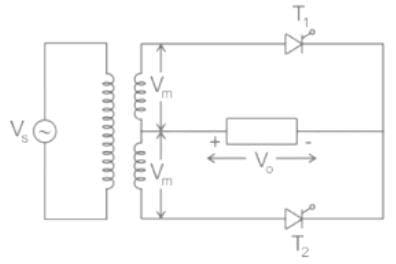Where,

Vs is the source voltage

Vm is the maximum voltage of the midpoint

Let α be the firing angle

V0 is the output voltage

When the thyristors are triggered with a firing angle 0 and π/2

Then the output waveform will be as follows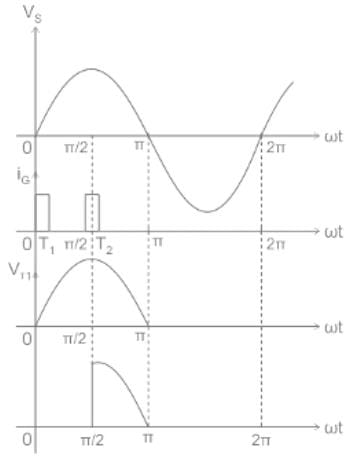Let the average output voltage of the circuit be Va

The value of source voltage is Vm sin ωt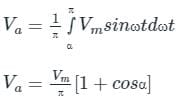Calculation:

Given,

α1 = 0, α2 = π/2

For the first thyristor, the average output voltage is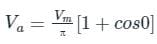Va = 2Vm / π

For the second thyristor, the average output voltage is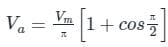Va = Vm / π

*Answer can only contain numeric values
Test: Single Phase Full wave mid point converters - Question 6

In a 12 phase full wave rectifier if source frequency is 30 Hz, then the ripple frequency will be_____Hz

Detailed Solution for Test: Single Phase Full wave mid point converters - Question 6

We know that

Ripple frequency = 2nf = 2 × 12 × 30 = 720 Hz

*Answer can only contain numeric values
Test: Single Phase Full wave mid point converters - Question 7

A single-phase AC supply is connected to a full wave rectifier through a transformer. The rectifier is required to supply an average DC output voltage of Vdc = 400 V to a resistive load of R = 10 Ω. The kVA rating of the transformer is __________ (in kVA)

Detailed Solution for Test: Single Phase Full wave mid point converters - Question 7

Concept:

In a full wave rectifier though a transformer,

Average output voltage, V0 = 2Vm

Average output current, I0 = V0/R

RMS value of output voltage Vor = Vs

RMS value of load current, Ior = Vs/R

Average value of diode current, Id = Im/2

RMS value of diode current, Idr = Ior

Peak value of diode current, Idm = √2 Ior

Power delivered to the load = Vor Ior

Input voltamperes = Vs Ior

Calculation:

Given that, DC output voltage (VDC) = 400 V

Average output voltage of rectifier (V0) = 400 V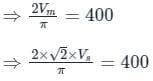⇒ Vs = 444.28 V

Load resistance (R) = 10 Ω

RMS value of load current,  Ior = 444.2810 = 44.428A

kVA rating of transformer = 444.28 × 44.428 = 19.738 kVA

Test: Single Phase Full wave mid point converters - Question 8

A single-phase full-wave controlled rectifier, operating at 120 V rms and 60 Hz ac supply, has a firing angle of 60°. The average value of its output voltage is

Detailed Solution for Test: Single Phase Full wave mid point converters - Question 8

Concept:

The average output voltage of the single-phase full-wave rectifier with a resistive load is given by
V0 = 2Vm/π cos α

Where,

Vm = peak value of source voltage

α = firing angle

Calculation:

Given that:

Source voltage Vs = 120 V rms

firing angle α = 600

From the source voltage peak value Vm = √2 × 120 V

The average output value of a single-phase full-wave rectifier is given by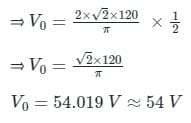Test: Single Phase Full wave mid point converters - Question 9

A 1ϕ full wave converter with RLE load wit R = 10Ω, L = 8mH and E = 150V. The AC voltage source is 230V, 50Hz for continuous conduction. Find the average value of load current for firing angle delay of 120°.

Detailed Solution for Test: Single Phase Full wave mid point converters - Question 9

Concept:

The average output voltage of a 1ϕ full wave converter is given by:

Case 1: α ≤ 90°

Vo(avg) = IoR + E

This is a motoring mode of operation of a rectifier.

Case 2: α ≥ 90°

Vo(avg) = IoR - E

This is a generating mode of operation of a rectifier.

In generating mode, the polarity of battery voltage gets reversed.

Calculation:

Given, α = 120°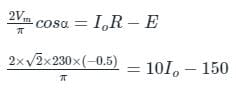Io = 4.64 A

Test: Single Phase Full wave mid point converters - Question 10

An AC voltage of maximum value equal to 100V is applied to a single-phase fully controlled bridge circuit. The peak inverse voltage rating of each SCR used will be ______.

Detailed Solution for Test: Single Phase Full wave mid point converters - Question 10

Concept:

Single-phase fully controlled bridge circuit: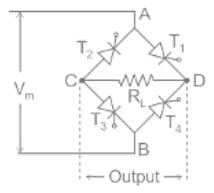• The bridge rectifier circuit is made of four SCRs T1, T2, T3, T4, and a load resistor RL.
• The four SCRs are connected in a closed-loop configuration to effectively convert the alternating current (AC) into Direct current (DC).
• The input signed is applied across terminals A and B and output DC signal is obtained across the load resistor RL connected across the terminals C and D.
• The four SCRs are arranged in such a way that only two SCRs conduct electricity during each half cycle.
• SCRs T1 and T3 are pairs that conduct electric current during the positive half cycle.
• Similarly, SCRs T2 and T4 conduct electric current during a negative half cycle.
• During the positive half cycle, SCRs T1 and T3 become forward biased while SCRs T2 and T4 become reverse biased.
• During the negative half-cycle, SCRs T2 and T4 become forward biased while SCRs T1 and T3 become reverse biased.
• The current flow across load resistor RL is the same during positing half-cycles and the negative half cycles.
• The output DC signal polarity may be either completely positive or negative.
• The bridge rectifier allows electric current during both positive and negative half cycles of the input AC signal.
• Peak inverse voltage is the maximum voltage that an SCR can withstand in a reverse bias condition.
• During the positive half cycle, SCRs T1 and T3 are conducting.
• Similarly, during the negative half cycle, SCR T2 and T3 are in the conducting stage while SCRs T1 and T3 are in a non-conducting state.

Calculation:

Given that

Vm = 100 V (AC signal)

PIV of bridge Rectifier =  PIV of SCR T1 and T3 = Vm = 100 V

Similarly, PIV of SCR T2 and T2 is Vm = 100 V.

i.e. PIV of each SCR T1, T2, T3, T4 is Vm = 100 V

## Power Electronics

5 videos|39 docs|63 tests
 Use Code STAYHOME200 and get INR 200 additional OFF Use Coupon Code
Information about Test: Single Phase Full wave mid point converters Page
In this test you can find the Exam questions for Test: Single Phase Full wave mid point converters solved & explained in the simplest way possible. Besides giving Questions and answers for Test: Single Phase Full wave mid point converters, EduRev gives you an ample number of Online tests for practice

## Power Electronics

5 videos|39 docs|63 tests